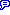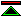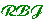# Definitions of Logic

## Overview:

 Some ways of defining "Logic" are introduced and their merits discussed.
 Introduction A bit of preliminary discussion about what we are looking for here. Evaluation Criteria How do we know when we have a good definition? Here are some criteria which we can use to assess candidates. methods, systems, truths are all involved, a definition can be approached through any one methods Logic can be defined as concerned with methods for reasoning. Logical systems are then formalisations of the proper methods and logical truths are those demonstrable by correct methods. systems logic can be defined as concerned with a certain class of logical systems, logical truths would then be defined in terms of their derivibility in the systems. truths Here certain kinds of truths are the definitive concern, systems and methods are then judged in terms of their ability to confirm this kind of truth.

## Introduction:

 A bit of preliminary discussion about what we are looking for here.
 The word "logic" It is not our concern here to give an exhaustive analysis of the use of the word "logic", or to make proposals about its use in general. For the reader interested in this topic a good dictionary is not a bad place to start. Defining Logics Methods for defining logical systems, and the definitions of particular system are of very great interest to us, but that's not what this page is about.
 Fundamentals It may be that there is some fundamental concept corresponding more or less to some of the more technical uses of the word logic, which will help us to build a better understanding of our world. It is this that we seek. In particular we are looking, as philosophers, for fundamental boundaries in methods of reasoning and in the kinds of truth which can be established by reason. Abstention One answer to this quest is possibly that there isn't anything fundamental, its all rather arbitrary.

## Evaluation Criteria:

 How do we know when we have a good definition? Here are some criteria which we can use to assess candidates.
 Fundamental, not ad. hoc. Definitions involving enumeration of a specific set of logical constants are a typical example of a definition which appears arbitrary and hence unsatisfactory, unless grounds were offered for the belief that the concept thus defined was of importance. Real Content Its easy to give definitions which have very little content at all. e.g. Quine's "Logic is the systematic study of the logical truths". No harm in this so long as you follow through and define logical truth. To get a good definition you need to work through several layers.
 Good Closure Properties Examples of desirable closure properties are closure under logical conjunction and closure under logical equivalence. So a definition (like that of Kant for analyticity) in terms of subject predicate sentences, which was not hedged to ensure closure would be unsatisfactory. Hedging with a phrase like "equivalent to" risks circularity! Well Definedness Settling for a form of words which doesn't really say anything is all too easy with these fundamental concepts. Meta-circular formal definitions can help if you can get that far.
 Relationship with Analytic and a priori Assuming you don't define logical truth directly in terms of analytic or a priori truth then the relationship with these is worth looking at. If there are substantial differences in extension of the three concepts then one is probably badly defined. If there are small difference its worth thinking about whether there really are two important but just slightly different concepts or whether one of them is not quite correctly formulated. Utility A sense of purpose may be helpful in sorting out good from bad definitions.

## Methods:

 A popular way of defining logic is as concerned with methods for correct reasoning.
 Copi According to Irving Copi (a philosopher), "Logic is the study of the methods and principles used to distinguish good (correct) from bad (incorrect) reasoning". He goes on to emphasise the distinction between logic and psychology. Shoenfield Joseph Shoenfield (a mathematician) opens his [] "Logic is the study of reasoning, and mathematical logic is the study of the type of reasoning done by mathematicians."
 advantages One advantage of this way of defining logic is that it fits the use of logic to derive false or contingent conclusions from premises which are not all logically necessary. A definition in terms of logical truth may seem to exclude this possibility. However, when we ask what it is that such a proof conclusively demonstrates we are not able simply to cite the conclusion, for we are assured only that the conclusion follows from the premises and hence would be true if they were. The deductive content is expressible as a single proposition (usually an entailment) and this we can expect to be logically true.
 disadvantages A disadvantage of identifying logic with methods is a possible difficulty in encompassing areas where there are no methods. If a definition of "logical truth" is desired (possibly in connection with establishing the correctness of the methods) some difficulty may be expected if starting from this point.

## Formal Systems:

 Logic may be defined as the study of formal logical systems. The results of logic would then be meta-theory about formal systems or formal theorems derived in such systems. This is not far off the mark for mathematical logic in academe.
 strict formalism Extreme formalists concern themselves exclusively with matters of syntax, regarding formal notations as devoid of meaning. From this point of view logic would be considered the study of formal "deductive" systems, and the question of logical truth may not arise. For a starting point in this kind of study try starting with What is Logic? (the un-philosophical version) and try to ignore the references to semantics. disadvantages: The disadvantages of this strict approach include some increased difficulty in accounting for the applicability of logic and mathematics, and some difficulty, in the light of Gödel's incompleteness results, in justifying interest in formal systems for arithmetic, which must fail in their purpose, without being able to mention that purpose.
 moderate formalism Mathematical logic as practised is not irrefrangibly hostile to semantics. Tarski showed how to render the semantics of first order logic, and a great deal of further work has been done since. It is therefore possible to define logic as the study of formal systems without abstaining from consideration of semantics. If this is done, detailed consideration of logical truth is brought into the subject rather than being part of the definition of the subject. In effect this amounts to the adoption of a definition of logical truth in terms of analyticity, where analyticity is defined in terms of meaning. This avoids both the problems mentioned in connection with strict formalist definitions of logic. A key merit of this approach is that it does not depend upon any arbitrary choice of formal system.

## Logical Truth:

 Logic may be defined in terms of a special class of truths which may be established by logical methods.
 logical truth The idea that there is some special collection of truths which should be called logical truths, among which number those truths which can be logically proven is widespread. There are however substantive disagreements about what these truths are, for example, about whether they include the truths of arithemetic. Furthermore, I know of no account of logical truth which encompasses all the truths which are obtainable within any proposed logical system. For example the results of inductive inference (meaning here scientific rather than mathematical induction) are not generally held to be logical truths.
 deductive or demonstrative It is therefore only reasonable to expect the idea of logical truth to encompass a subset of the logics which have been formulated. A first indication of the kinds of logic which we might expect to be encompassed by a definition of logical truth is deductive or demonstrative logic, the primary feature of which logics is that we have generally reasonable grounds for believing that they are sound in the sense that only true conclusions are derivable from true premises.
 defining logic Quine has defined logic(in [Quine70]) as "the systematic study of the logical truths". Our own preferred definition is that logic "is the study of necessary truths and of systematic ways of expressing and establishing such truths". Clearly either one of these does not take use very far and the proof of the pudding is not to be had until considerable further elaboration has been supplied.©created 1997/06/02 modified 1998/07/23